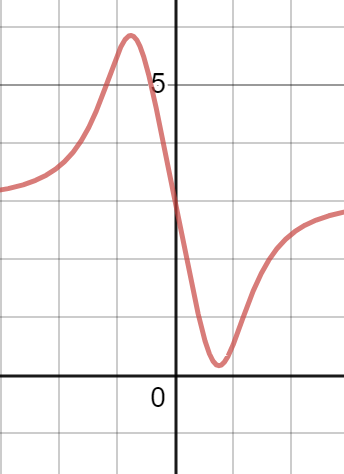Consider the function F(x)=\frac{3x^{4}-5x+3}{x^{4}+1} Find: Domain Vertical,horizontal,or slant...

Question:

Consider the function {eq}\displaystyle F(x)=\frac{3x^{4} - 5x + 3}{x^{4} + 1} {/eq}

Find: Domain Vertical,horizontal,or slant asymptotes x-intercept(s) y-itercept(s).

Horizontal Asymptotes:

The function defined by {eq}\displaystyle{ \begin{align} f(x) \end{align} } {/eq} has at most two horizontal asymptotes. The horizontal asymptote to the left is given by

{eq}\displaystyle{ \begin{align} y=\lim{x\to -\infty} f(x) \end{align} } {/eq}

if it exists. The horizontal asymptote to the right is given by

{eq}\displaystyle{ \begin{align} y=\lim{x\to \infty} f(x) \end{align} } {/eq}

if it exists.

These asymptotes may or may not exist, and they may be equivalent expressions of the same asymptote.

Let

{eq}\displaystyle{ \begin{align} F(x)=\frac{3x^4-5x+3}{x^4+1} \end{align} } {/eq}

The domain of the function is all real numbers, as the denominator will never be zero.

Since the domain is {eq}\displaystyle{ \begin{align} \left( -\infty , \infty \right) \end{align} } {/eq}, there are no vertical asymptotes.

The limits at infinity are

{eq}\displaystyle{ \begin{align} \lim_{x \to -\infty} \frac{3x^4-5x+3}{x^4+1} = \frac{3}{1} = 3\\ \lim_{x \to \infty} \frac{3x^4-5x+3}{x^4+1} = \frac{3}{1} = 3\\ \end{align} } {/eq}

So, there is one horizontal asymptote, and the graph approaches it both to the left and the right. It is {eq}\displaystyle{ \begin{align} y=3 \end{align} } {/eq}

The intercepts are more complicated. The graph is shown below.The graph approaches the horizontal line {eq}\displaystyle{ \begin{align} y=3 \end{align} } {/eq} to the left and the right of the graph shown, so there are no {eq}\displaystyle{ \begin{align} x \end{align} } {/eq}-intercepts.

The {eq}\displaystyle{ \begin{align} y \end{align} } {/eq}-intercept is given when {eq}\displaystyle{ \begin{align} x=0 \end{align} } {/eq}.

{eq}\displaystyle{ \begin{align} f(0)=\frac{3(0)^4-5(0)+3}{(0)^4+1}=3 \end{align} } {/eq}

So, the {eq}\displaystyle{ \begin{align} y \end{align} } {/eq}-intercept is {eq}\displaystyle{ \begin{align} (0,3) \end{align} } {/eq}.